Courses

# Doc: Collisions Class 11 Notes | EduRev

## Class 11 : Doc: Collisions Class 11 Notes | EduRev

The document Doc: Collisions Class 11 Notes | EduRev is a part of the Class 11 Course Physics Class 11.
All you need of Class 11 at this link: Class 11

Collisions

Collision between two or more particles is the interaction for a short interval of time in which they apply relatively strong forces on each other.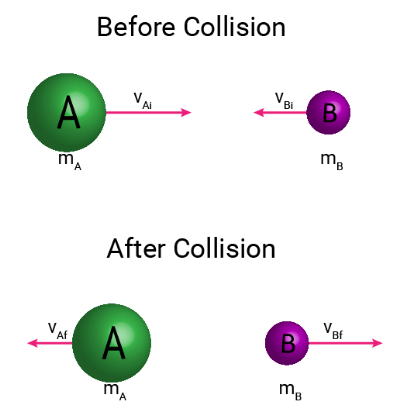In a collision physical contact of two bodies is not necessary. there are two types of collisions:

1. Elastic collision

The collision in which both the momentum and the kinetic energy of the system remains conserved are called elastic collisions.

In an elastic collision all the involved forces are conservative forces.

Total energy remains conserved.

2. Inelastic collision

The collision in which only the momentum remains conserved but kinetic energy does not remain conserved are called inelastic collisions.

In an inelastic collision some or all the involved forces are non-conservative forces.

Total energy of the system remains conserved.

If after the collision two bodies stick to each other, then the collision is said to be perfectly inelastic.

Coefficient of Restitution or Resilience

The ratio of relative velocity of separation after collision to the velocity of approach before collision is called coefficient of restitution resilience.

It is represented by e and it depends upon the material of the collidingI bodies.

For a perfectly elastic collision, e = 1

For a perfectly inelastic collision, e = 0

For all other collisions, 0 < e < 1

One-Dimensional or Head-on Collision

If the initial and final velocities of colliding bodies lie along the same line, then the collision is called one dimensional or head-on collision.

Inelastic One Dimensional Collision

Applying Newton’s experimental law, we have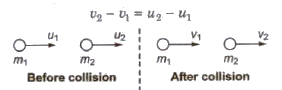Velocities after collision

v1 = (m1 – m2) u1 + 2m2u2 / (m1 + m2)

and v2 = (m2 – m1) u2 + 2m1u1 / (m1 + m2)

When masses of two colliding bodies are equal, then after the collision, the bodies exchange their velocities.

v1 = u2 and v2 = u1

If the second body of same mass (m1 = m2) is at rest, then after collision first body comes to rest and second body starts moving with the initial velocity of the first body.

v1 = 0 and v2 = u1

If a light body of mass m1 collides with a very heavy body of mass m2 at rest, then after a collision.

v1 = – u1 and v2 = 0

It means the light body will be rebound with its own velocity and heavy body will continue to be at rest.

If a very heavy body of mass m1 collides with a light body of mass m2(m1 > > m21) at rest, then after collision

v1 = u1 and v2 = 2u1

In Inelastic One Dimensional Collision

Loss of kinetic energy

ΔE = m1m2 / 2(m1 + m2) (u1 – u2)2 (1 – e2)

In Perfectly Inelastic One Dimensional Collision

Velocity of separation after collision = 0.

Loss of kinetic energy = m1m2 (u1 – u2)2 / 2(m1 + m2)

If a body is dropped from a height ho and it strikes the ground with velocity vo and after inelastic collision, it rebounds with velocity v1 and rises to a height h1, then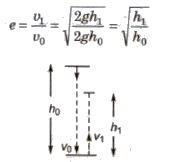If after n collisions with the ground, the body rebounds with a velocity vn and rises to a height hn then

en = vn / vo = √hn / ho

Two-Dimensional or Oblique Collision

If the initial and final velocities of colliding bodies do not lie along the same line, then the collision is called two dimensional or oblique Collision.

In horizontal direction,

m1u1 cos α+ m2u2 cos α2= m1v1 cos β1 + m2v2 cos β2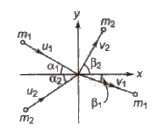In vertical direction.

m1u1 sin α1 – m2u2 sin α2 = m1u1 sin β1 – m2u2 sin β2

If m1 = m2 and α1 + α2 = 90°

then β1 + β2 = 90°

If a particle A of mass m1 moving along z-axis with a speed u makes an elastic collision with another stationary body B of mass m2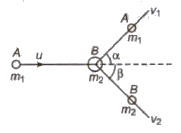From conservation law of momentum

m1u = m1v1 cos α + m2v2 cos β

sin β2v2 sin α – m1v1O = m

Offer running on EduRev: Apply code STAYHOME200 to get INR 200 off on our premium plan EduRev Infinity!

## Physics Class 11

75 videos|222 docs|152 tests

,

,

,

,

,

,

,

,

,

,

,

,

,

,

,

,

,

,

,

,

,

;